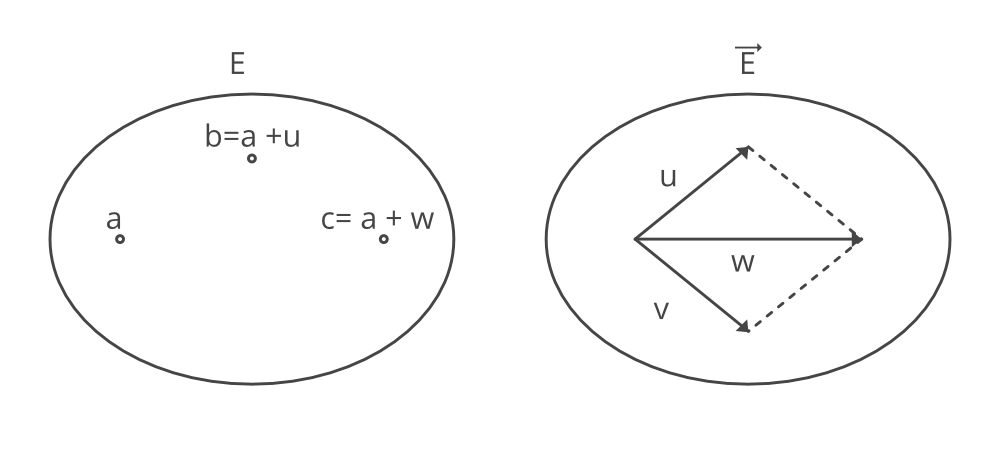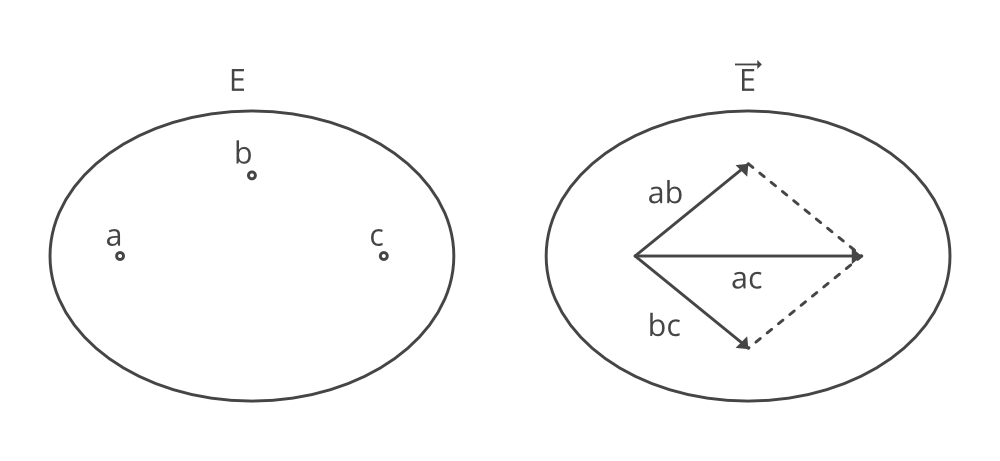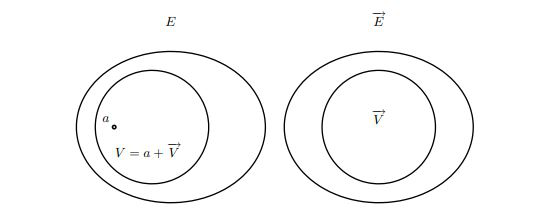GeeksforGeeks App
Open AppBrowser
Continue

## Related Articles

### Affine spacesAffine space

Affine space is the set E with vector space \vec{E} and a transitive and free action of the additive \vec{E} on set E. The elements of space A are called points. The vector space \vec{E} that is associated with affine space is known as free vectors and the action +: E * \vec{E} \rightarrow E satisfying the following conditions:

1. Right Identity: a + 0 = a2. Associativity:3. For any two points a,b \epsilon E, there is unique u such that :where u \epsilon \vec{E} and can be denoted ab or \vec{ab} or sometimes b-a. Therefore , we can write above equation### Example:

. Consider the subset L ofconsisting of all points (x, y) satisfying the equation:L is the line having slope -1 passing through the point (1,0) and (0,1). The line L can be an affine space by defining the action +: L * R \rightarrow L of R on L defined such that every point (x, 1-x) on L and any u \epsilon R.Now, for any two points a =(a_1, 1- a_1) and b = (b_1, 1-b_1) on L, the unique vector u \epsilon R such that b = a+u is u = b_1 – a_1. Note that vector space R isomorphic to the line of equation x + y = 0 passing through the origin.

### Chasles’s IdentityGiven any three points, since c = a + ac, b = a + ab, and c = b + bc, we getBy applying the above properties 2 and 3,THe above equation is known as chasles Identity. Since

a = a + aa

and by using property 1 we,get

a = a+ 0

Thus, by using property 3, we get:Replacing a in place of c in Chasles Identity, we get:

ba =-ab

Now, For 4 points a,b,c,d \epsilon E. The chasles identity can be given as:

## Affine combinations/ Barycentres

Similar to linear combination in linear algebra, the corresponding concept in affine geometry is that of an affine combination, also called a barycenter

Consider 2-dimensional space as an affine space, with origin O= (0,0) and basis vectors (1,0) and (0,1). Given any two points a =(a1, a2) and b =(b1, b2) there can be a natural combination such that \lambda a+ \mu b or:when a = (-1, -1) and b = (2, 2), thus a+b can be given as: c = (1,1).

Now, consider the new coordinate system with respect to the origin c = (1, 1). Now, the co-ordinates of a = (-2, -2), the co-ordinates of b are (1, 1), and the point of d = (-1, -1). However, point d is identical to the origin O = (0, 0) of the first coordinate system.

Thus, a + b corresponds to two different points depending on which coordinate system is used for its computation. This means that we need extra conditions are required for affine computations. It turns out the scalars sum up 1. This helps us define the Barycentres

For any family of points (a_i)_{i\epsilon I} in  E, for any family of scalars such thatand for any, the pointis called the barycentres of the points a_i assigned the weightsand is denoted by:Barycentres is conveniently denoted by the notations, whereasis a point andis called a scalar.

### Affine SubspaceV is the affine subspace ofin the direction ofGiven an affine space, a subset V of E is an affine subspace of, if for every family of weighted pointsin V such that, the barycenterbelongs to V .

### References:

My Personal Notes arrow_drop_up